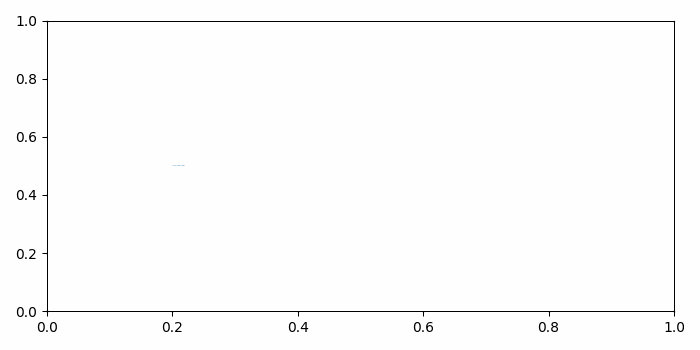# How to animate text in Matplotlib?

MatplotlibPythonData Visualization

To animate text in matplotlib, we can take the following steps −

• Import "animation" package from matplotlib.
• Set the figure size and adjust the padding between and around the subplots.
• Create a new figure or activate an existing figure.
• Add an 'ax' to the figure as part of a subplot arrangement.
• Initialize a variable "text" to hold a string.
• Add text to the axes at x=0.20 and y=0.50.
• Make a list of colors.
• Make an animation by repeatedly calling a function *animate*, where size of text is increased and color is changed.
• To display the figure, use show() method.

## Example

from matplotlib import animation
import matplotlib.pyplot as plt

plt.rcParams["figure.figsize"] = [7.00, 3.50]
plt.rcParams["figure.autolayout"] = True

fig = plt.figure()
text = 'You are welcome!'
txt = ax.text(.20, .5, text, fontsize=15)

colors = ['#1f77b4', '#ff7f0e', '#2ca02c', '#d62728', '#9467bd', '#8c564b', '#e377c2',    '#7f7f7f', '#bcbd22', '#17becf']

def animate(num):
txt.set_fontsize(num * 2 + num)
txt.set_color(colors[num % len(colors)])
return txt,

anim = animation.FuncAnimation(fig, animate, frames=len(text) - 1, blit=True)

plt.show()

## Output

It will produce the following output